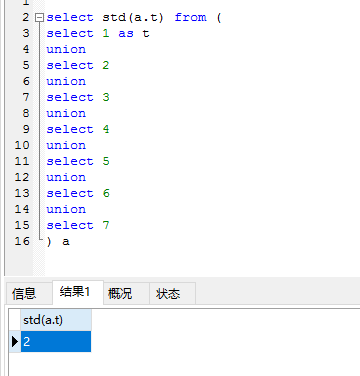分布式计算 标准差，信度

A机器计算 (1、2、3、4）

B机器计算 （5、6、7）

((110+30)/(4+3))-((10+18)/(4+3))²

val rdd1 = sc.makeRDD(Array(("A",1),("A",2),("A",3),("A",4),("A",5),("A",6),("A",7)))

rdd1.combineByKey(
(v : Int) => List(v,v*v,1),
(c : List[Int], v : Int) => List(c.apply(0)+v,(c.apply(1)+v*v),c.apply(2)+1),
(c1 : List[Int], c2 : List[Int]) => List(c1.apply(0)+c1.apply(0),c1.apply(1)+c1.apply(1),c1.apply(2)+c1.apply(2))
).mapValues(x => sqrt((x.apply(1)/x.apply(2)) - (x.apply(0)/x.apply(2))*(x.apply(0)/x.apply(2)))).collect().foreach(println)
def sqrtIter(guess: Double, x: Double): Double =
if (isGoodEnough(guess, x))
guess
else
sqrtIter((guess + x / guess)/2, x)

// 判断解是否满足要求
def isGoodEnough(guess: Double, x: Double) =
abs(guess * guess - x)< 0.0001

// 辅助函数，求绝对值
def abs(x: Double) =
if (x < 0) -x else x

// 目标函数
def sqrt(x: Double): Double =
sqrtIter(1, x)posted @ 2018-04-27 18:10  George_sz  Views(529)  Comments(0Edit  收藏  举报